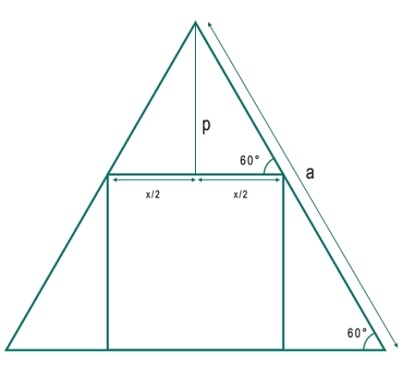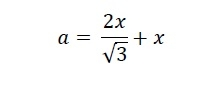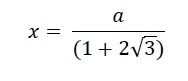# Biggest Square that can be inscribed within an Equilateral triangle?

Here we will see the area of the biggest square that can be inscribed in an equilateral triangle. The side of the triangle is ‘a’ and the side of the square is x.The side of the triangle ‘a’ is −So x is −## Example

#include <iostream>
#include <cmath>
using namespace std;
float areaSquare(float a) { //a is side of triangle
if (a < 0 ) //if a is negative, then this is invalid
return -1;
float area = a / (1 + 2/sqrt(3));
return area;
}
int main() {
float a = 7;
cout << "Area of Rectangle: " << areaSquare(a);
}

## Output

Area of Rectangle: 3.24871